RS Aggarwal Solutions Class 10 Ex 18C

RS Aggarwal Class 10 Ex 18C Chapter 18

Q.1: A sector is cut from a circle of radius 21 cm. The angle of the sector is $150^{\circ}$. Find the length of the arc and the area of the sector.

Sol:

Given:

Angle of sector = $150^{\circ}$

Now,

Length of arc = $= \frac{2 \pi r \theta }{360}$

$= \frac{2 \times \frac{22}{7} \times 21 \times 150 }{360}$

= 55 cm

Area of the sector = $= \frac{\pi r^{2} \theta }{360}$

$= \frac{\frac{22}{7} \times 21\times 21 \times 150 }{360}$ = 577.5 $cm^{2}$

Q.2: The area of the sector of a circle of radius 10.5 cm is 69.3 $cm^{2}$. Find the central angle of the sector.

Sol:

Given

Area of the sector = 63 $cm^{2}$

Now,

Area of the sector = $= \frac{\pi r^{2} \theta }{360}$

$\Rightarrow 69.3 = \frac{\frac{22}{7} \times 10.5 \times 10.5 \times \theta }{360}$

$\Rightarrow \theta =\frac{69.3 \times 7 \times 360}{22 \times 10.5 \times 10.5}$

$\Rightarrow \theta = 72^{\circ}$

Therefore, Central angle of the sector = $72^{\circ}$

Q.3 The length of an arc of a circle, subtending an angle of $54^{\circ}$ at the centre is 165 cm. Calculate the radius, circumference and area of the circle.

Sol:

Length of the arc = 16.5 cm

$\theta = 54^{\circ}$

Circumference = ?

We know:

Length of the arc = $= \frac{2 \pi r \theta }{360}$

$\Rightarrow 16.5 = \frac{2 \times \frac{22}{7} \times r \times 54}{360}$

$\Rightarrow r = \frac{16.5 \times 360 \times 7}{44 \times 54}$

$\Rightarrow r = 17.5$ cm

$c = 2 \pi r$

$c = 2 \times \frac{22}{7} \times 17.5$ = 110 cm

Circumference = 110 cm

Now,

Area of the circle = $= \pi r^{2}$

= $\frac{22}{7} \times 17.5 \times 17.5$ = $962.5 \; cm^{2}$

Q.4: The radius of a circle with center O is 7 cm. Two radii OA and OB are drawn at right angles to each other. Find the areas of minor and major segments.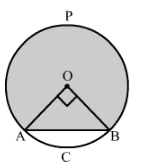Area of minor segment = Area of sector AOBC – Area of right triangle AOB

$= \frac{90}{360} \pi (OA)^{2} – \frac{1}{2} \times OA \times OB$

$= \frac{1}{4} \times \frac{22}{7} \times (7)^{2} – \frac{1}{2} \times 7 \times 7$

$= 38.5 – 24.5$

= 14 $cm^{2}$

Area of major segment APB = Area of circle – Area of minor segment

$\pi (OA)^{2} – 14$

$= \frac{22}{7} \times (7)^{2} – 14$

$= 154 – 14$

$= 140 \; cm^{2}$

Hence, the area of major segment is $= 140 \; cm^{2}$

Q.5: Find the length of the arcs cut off from a circle of radius 12 cm by a chord 12 cm long. Also, find the area of the minor segment.   ($\pi = 3.14 \; and \; \sqrt{3} = 1.73$)

Sol: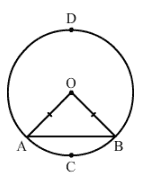Let AB be the chord. Joining A and B to O, we get an equilateral triangle OAB.

Thus we have:

$\angle O = \angle A = \angle B = 60^{\circ}$

Let the arc ACB:

$= 2 \pi \times 12 \times \frac{60}{360}$ = $4\pi$ = 12.56 cm

Circumference of the circle – length of the arc ACB

= $2\pi \times 12 – 4\pi$$= 20 \pi$ = 62.80 cm

Now, Area of the minor segment:

Area of the sector – Area of the triangle

$= \left [ \pi \times (12)^{2} \times \frac{60}{360} – \frac{\sqrt{3}}{4} \times (12)^{2}\right ]$ = 13.08 $cm^{2}$

Q.6: A chord 10 cm long is drawn in a circle whose radius is $5 \sqrt{2} \; cm$. Find the areas of both the segments.  [Take $\pi = 3.14$ ]

Sol:

Let O be the centre of the circle and AB be the chord.

Consider $\bigtriangleup AOB$

OA = OB = $5\sqrt{2} \; cm$

$OA^{2} + OB^{2} = 50+50 =100$

Now,

$\sqrt{100}= 10 \; cm = AB$

Thus $\bigtriangleup AOB$ is a right isosceles triangle.

Thus, we have :

Area of $\bigtriangleup OAB = \frac{1}{2} \times 5\sqrt{2} \times 5\sqrt{2} = 25 cm^{2}$

Area of the minor segment = Area of the sector – Area of the triangle

$= \left ( \frac{90}{360} \times \pi \times (5\sqrt{2})^{2} \right ) – 25$ = $14.25 \; cm^{2}$

Area of the major segment = Area of the circle – Area of the minor segment

= $\pi \times (5\sqrt{2})^{2} – 14.25$ = $14.75 \; cm^{2}$

Q.7: Find the area of both the segments of a circle of radius 42 cm with central angle $120^{\circ}$.   ($\sin 120^{\circ} = \frac{\sqrt{3}}{2} \; and \; \sqrt{3} = 1.73$ )

Sol:

Area of the minor sector = $\frac{120}{360} \times \pi \times 42 \times 42$

= $\frac{1}{3} \times \pi \times 42 \times 42$ = 1848 $cm^{2}$

Area of the triangle = $\frac{1}{2} R^{2}\sin \theta$

Here, R is the measure of the equal sides  of the isosceles triangle and $\theta$ is the angle enclosed by the equal sides.

Thus, we have

$\frac{1}{2} \times 42 \times 42 \times \sin (120^{\circ})$

= 762.93 $cm^{2}$

Area of the minor segment = Area of the sector – Area of the triangle

= 1848 – 762.93 = 1085.07 $cm^{2}$

Area of the major segment = Area of the circle – Area of the minor segment

= $(\pi \times 42 \times 42) – 1085.07$

= 5544 – 108.07 = 4458.93 $cm^{2}$

Q.8: A chord of a circle of radius 30 cm makes an angle of $60^{\circ}$ at the centre of the circle. Find the areas of the minor and major segments.   [Take $\pi = 3.14 \; and \; \sqrt{3} = 1.732$ ]

Sol: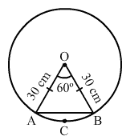Let the chord be AB. The ends of the chord are connected to the centre of the circle O to give the triangle OAB.

OAB is an isosceles triangle. The angle at the centre is $60^{\circ}$

Area of the triangle = $\frac{1}{2} \times (30)^{2} \sin 60^{\circ} = 450 \times \frac{\sqrt{3}}{2} = 389.25 \; cm^{2}$

Area of the sector OACBO = $\frac{60}{360} \times \pi \times 30 \times 30 = 150 \pi = 471 \; cm^{2}$

Area of the minor segment = Area of the segment – Area of the triangle = 471 – 389.25 = 81.75 $cm^{2}$

Area of the major segment = Area of the circle – Area of the minor segment

= $(\pi \times 30 \times 30) – 81.29$ = 2744.71 $cm^{2}$

Q.9: In a circle of radius 10.5 cm, the major arc is one-fifth of the major arc. Find the area of the sector corresponding to the major arc.

Sol:

Let the length of the major arc be x cm.

Radius of the circle = 10.5 cm

Therefore, Length of the minor arc = $\frac{x}{5} \; cm$

Circumference = $x + \frac{x}{5} = \frac{6x}{5} \; cm$

Using the given data, we get :

$\frac{6x}{5} = 2 \times \frac{22}{7} \times \frac{21}{2}$

$\Rightarrow \frac{6x}{5} = 66$

$\Rightarrow x = 55$

Therefore, Area of the sector corresponding to the major arc = $\frac{1}{2} \times 55 \times \frac{21}{2} = 288.75 \; cm^{2}$

Q.10: The short and long hands of a clock are 4 cm and 6 cm respectively. Find the sum of distances travelled by their tips in 2 days.     [Take $\pi = 3.14$]

Sol:

In 2 days, the short hand will complete 4 rounds.

Length of the short hand = 4 cm

Distance covered by the short hand = $= 4 \times 2\pi \times 4 = 32 \pi$ cm

In the same 2 days, the long hand will complete 48 rounds.

Length of the long hand = 6 cm

Distance covered by the long hand = $= 48 \times 2\pi \times 4 = 576 \pi$ cm

Therefore, Total distance covered by both the hands = Distance covered by the short hand + Distance covered by the long hand

= $32 \pi +576 \pi$ = $608 \pi$ = $608 \times 3.14$ = $1909.12$ cm

Q.11: Find the area of a quadrant of a circle whose circumference is 88 cm.

Sol:

Let the radius of the circle be r.

Now,

Circumference = 88 cm

$\Rightarrow 2 \pi r = 88$

$\Rightarrow r = 14$ cm

Now,

Area of quadrant $= \frac{1}{4} \pi r^{2} = \frac{1}{4} \times \frac{22}{7} \times (14)^{2} = 154 \; cm^{2}$

Hence, the area of the quadrant is $15 cm^{2}$.

Q.12: A rope by which a cow is tethered is increased from 16 cm to 23 cm. How many additional grounds does it have now to graze?

Sol: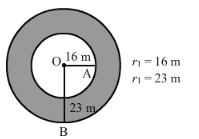$r_{1} = 16 \; cm$

$r_{2} = 23 \; cm$

Amount of additional ground available = Area of the bigger circle – Area of the smaller circle

$= \pi (r_{1}^{2} – r_{2}^{2})$

$= \pi (23^{2} – 16^{2})$$= \pi (23 + 16) (23 – 16)$$= 858 \; m^{2}$

Q.13: A horse is placed for grazing inside a rectangular field 70 m by 52 m. It is tethered to one corner by a rope 21 m long. On how much area can it graze? How much area is left unglazed?

Sol:

The shaded portion shows the part of the field the horse can graze.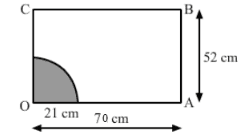Area of the grazed field = Area of the quadrant OPQ

$= \frac{1}{4}$ of the circle having radius OP.

$= \frac{1}{4} \pi r^{2}$

$= \frac{1}{4} \times \frac{22}{7} \times 21 \times 21$

= 346.5 $m^{2}$

Total area of the field = $70 \times 52 = 3640 \; m^{2}$

Area left ungrazed  = Area of the field – Area of the grazed field

= 3640 – 346.5 = 3293.5 $m^{2}$

Q.14: A horse is tethered to one corner of a field which is in the shape of an equilateral triangle of side 12 m. If the length of the rope is 7 m, find the area of the field which the horse cannot graze. Take $\sqrt{3} = 1.732$. Write the answer correct to 2 decimal places.

Sol:

Side of the equilateral triangle = 12 m

Area of the equilateral triangle = $\frac{\sqrt{3}}{4} \times (Side)^{2}$

$\frac{\sqrt{3}}{4} \times 12 \times 12$

= 62.28 $m^{2}$

Length of the rope = 7 m

Area of the field that horse can graze is the area of the sector of radius 7 m. Als, the angle subtended at the centre is $60^{\circ}$

$\frac{\theta }{360} \times \frac{22}{7} \times (7)^{2}$ = 25.67 $m^{2}$

Area of the field that horse cannot graze = Area of the equilateral triangle – Area of the field the horse can graze = 62.28 – 25.67 = 36.61 $m^{2}$

Q.15: Four cows are tethered at the four corners of a square field of side 50 m such that each can graze the maximum unshared area. What area will be left unglazed?    [Take $\pi = 3.14$ ]

Sol: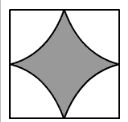Each cow can graze a region that cannot be accessed by the cows.

Therefore, Radius of the region grazed by each cow $= \frac{50}{2} = 25$

Area that each cow grazes = $\frac{1}{4} \pi r^{2}$

$\frac{1}{4} \times 3.14 \times 25 \times 25$

$= 490.625 cm^{2}$

Total area grazed = $4\times 490.625 = 1963.49 \; m^{2}$

Area of the square = $(Side)^{2}$

$=(50)^{2}$ = 2500 $cm^{2}$

Now,

Area left ungrazed = Area of the square – Grazed area = 2500 – 1963.49 = 563.51 $m^{2}$

Q.16: In the given figure, OPQR is a rhombus, three of whose vertices lie on a circle with centre O. If the area of the rhombus is $32\sqrt{3} \; cm^{2}$, find the radius of the circle.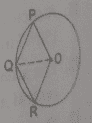Sol:

In a rhombus, all sides are congruent to each other.

Thus, we have:

OP = PQ = QR = RO

Now, consider $\bigtriangleup QOP$

OQ = OP (Both the radii)

Therefore, $\bigtriangleup QOP$ is equilateral.

Similarly, $\bigtriangleup QOR$ is equilateral and $\bigtriangleup QOP \cong \bigtriangleup QOR$

Ar. (QROP) = Ar. ($\bigtriangleup QOP$) + Ar. ($\bigtriangleup QOR$) = 2 Ar. ($\bigtriangleup QOP$)

Ar. $(\bigtriangleup QOP) = \frac{1}{2} \times 32 \sqrt{3} = 16 \sqrt{3}$

Or,

$16\sqrt{3} = \frac{\sqrt{3}}{4} s^{2}$   ( where s is the side of the rhombus )

Or,

$s^{2} = 16 \times 4 = 64$

$s = 8$ cm

$Therefore, OQ = 8$ cm

Hence, the radius of the circle is 8 cm.

Practise This Question

The graph given below is that of 2xy=0